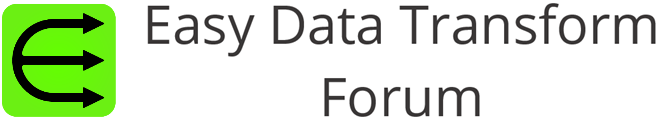# Round with Decimal Places

The current Round action on Calculate operation will perform rounding to the whole number only. I would like the option to specify the decimal places to round. So that if my data is 12.345, I can round this to 12.35 in one step.

(Yes, you can multiply the original number by 100, then use the current round feature, and then divide by 100. That is a lot of steps to perform something that would appear to be simple.)

As a nice to have, it would be good to offer “Round”, “Round Up”, and “Round Down”.

The Num Format transform rounds any which way. Except for the Round up or Round down.

1 Like

AS @DanFeliciano says, you can use the Num format to round to a certain number of decimal places. Also you can use floor, ceil and round in the Calculate transform.

‘Rounding’ is a deceptively complex topic. Even IEEE 754 has material problems in some applications, such as finance. Alternatives such as BigDecimal or fractional number representations also have issues, and are much more complex to implement. Binary 128 solves some of the issues, but is not implemented widely as yet, AFAIK.

There are many applications where rounded number appears to be fine, but in fact leave tiny residuals which accumulate over time and then generate a wrong result.

It’s maybe not a big issue for EDT users, but it does need some thought about the possible side effects.

We are generally using 64 bit variables for floating point operations in Easy Data Transform. If you really need more than 64 bit precision, then Easy Data Transform probably isn’t the right solution for you.

Not intended as a critique of EDT (which is wonderful…) It was just a passing general point about rounding being more complex than one might think. EDT itself won’t have problems with (for example) leaving accumulating fractional amounts as it’s not going to be preforming the repeated update scenarios which may rise to same.

Sorry if that came across as a bit abrupt.

Indeed rounding and floating point numbers is a more complex problem than most people realize.